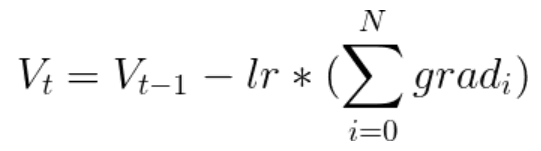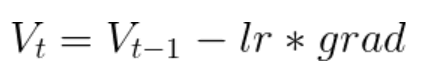×

Search anything:

# Gradient Accumulation [+ code in PyTorch]

#### Deep Learning Machine Learning (ML)Gradient Accumulation is an optimization technique that is used for training large Neural Networks on GPU and help reduce memory requirements and resolve Out-of-Memory OOM errors while training. We have explained the concept along with Pytorch code.

1. Background on training Neural Networks
2. What is the problem in this training process?
6. Concluding Note

Following table summarizes the concept of Gradient Accumulation:

What is it?It is an optimization technique to reduce memory requirements in training Neural Networks on GPU.
Used in:Training phase of Neural Networks
Basic IdeaSplit batch into smaller batches and add up loss gradient without updation.
Issued solvedOut Of Memory (OOM) error while training Neural Network on GPU.
InventorsJoeri R. Hermans, Gerasimos Spanakis and Rico Möckel
Inventor AffiliationLiège University, Belgium and Maastricht University, The Netherlands
Designed2017
Published inJMLR: Workshop and Conference Proceedings
Weight update formula with Gradient Accumulation## Background on training Neural Networks

Following is the general process of training a Neural Network on GPU:

1. We train a Neural Network on a training dataset and use a fixed batch size which represents the size of a random sample of the training dataset to be used for training.
2. We select a random sample of batch size B.
3. Neural Network undergoes forward pass using the current sample and generates predictions.
4. The generated prediction is compared with the actual result and the difference/ loss is computed. Different optimizers are used in this process such as SGD or Adam Optimizer.
5. The gradient of the loss is calculated with respect to all parameters (such as weights and bias) of the Neural Network during the backward pass and the parameters are updated based on the calculated gradient. Another parameter is used known as learning rate (LR) which determines the rate of update in the parameters.

The formula of updating the parameters is as follows:Figure 1: Weight Update Formula for SGD

The formula is for SGD optimizer. The exact formula will depend on the optimizer you use but the steps will remain the same.

## What is the problem in this training process?

The problem is the batch size.

Neural Networks are growing in size and for Image based applications, the dataset involve high resolution images which are memory intensive as well. GPUs have limited memory due to which depending on the GPU you are using, the training process may fail if you use a higher batch size than supported by your GPU.

If can be that a batch size of 8 will be the maximum limit of your GPU. Yes, training is possible in this case but it will consume a significant amount of time as smaller batch sizes will require more iterations to cover the training dataset and maintain overall accuracy.

One of the optimizations developed to address this issue is known as "Gradient Accumulation".

Gradient Accumulation is an optimization technique for training a Neural Network to address the large memory requirement issue. This technique is applicable for every loss function including SGD and Adam Optimizer.

The steps are:

• Original batch size is B which gives Out Of Memory (OOM) error while training on GPU.
• The batch size B is split into N smaller batches each with B/N size. The size (B/N) works fine on the GPU system.
• The evaluation, loss computation and calculation of loss gradient of each batch is done without updating the parameters.
• After processing all small batches, the parameters are updated based on the added up loss gradient.

In short, the idea of Gradient Accumulation is:

The batch size is split into smaller batch sizes and the loss gradient for each smaller batch is summed up (accumulated) without updating the parameters. After adding up loss gradient of each smaller batch, the parameters are updated.Figure 2: Weight Update Formula with Gradient Accumulation for SGD

This optimization was introduced in the research paper titled "Accumulated Gradient Normalization" by Joeri R. Hermans, Gerasimos Spanakis and Rico Möckel from Liège University, Belgium and Maastricht University, The Netherlands. The paper was published in 2017 at JMLR: Workshop and Conference Proceedings.

Gradient Accumulation is implemented in modern Deep Learning frameworks like PyTorch.

Following is the Python code implementing Gradient Accumulation in PyTorch:

``````# With Gradient Accumulation
total_loss = 0
num_accumulation_steps = 5

for i, (inputs, labels) in enumerate(training_set):
# Forward pass
predictions = model(inputs)
# Compute loss function
loss = loss_function(predictions, labels)

# accumulate loss for num_accumulation_steps (5)
if (i+1) % num_accumulation_steps != 0:
total_loss = loss + total_loss
# done once every num_accumulation_steps (5) steps
elif (i+1) % num_accumulation_steps == 0:
# Normalize loss
total_loss = (total_loss + loss ) / num_accumulation_steps
# Backward pass
loss.backward()
# parameters updated
optimizer.step()
total_loss=0
evaluate_model()
``````

If Gradient accumulation is not supported, the above code using PyTorch in Python will be as follows:

``````# Without Gradient Accumulation
for i, (inputs, labels) in enumerate(training_set):
# Forward pass
predictions = model(inputs)
# Compute loss function
loss = loss_function(predictions, labels)
# Backward pass
loss.backward()
# parameters updated
optimizer.step()
evaluate_model()
``````

Gradient Accumulation provides a better direction towards local minima compared to First Order Gradients (without Gradient Accumulation). Hence, the training process reaches the optimal parameters quickly.

There is no accuracy loss in using Gradient Accumulation.

## Concluding Note

With this article at OpenGenus, you must have the complete idea of this important optimization for training Neural Network: Gradient Accumulation.

Training is the most computational intensive operation for Neural Networks and has been made possible mainly due to recent hardware process like GPUs. Still limitation is there and optimizations like Gradient Accumulation help towards this.#### Jonathan Buss

Associate Professor at University of Waterloo | BSc in Computing from California Institute of Technology, PhD from Massachusetts Institute of Technology (MIT)

Gradient Accumulation [+ code in PyTorch]